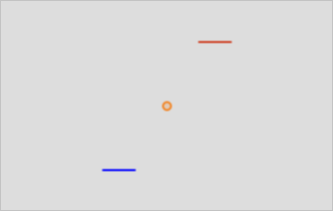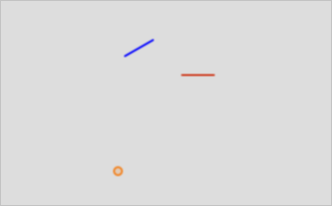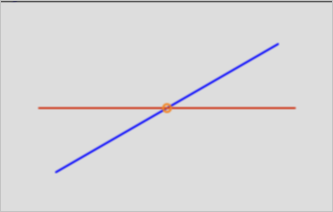This topic describes the ST_Rotate function. This function rotates a geometry object by specific radians in the anticlockwise direction.

## Syntax

``````geometry  ST_Rotate(geometry  geomA , float  rotRadians);
geometry  ST_Rotate(geometry  geomA , float  rotRadians , float  x0 , float  y0);
geometry  ST_Rotate(geometry  geomA , float  rotRadians , geometry  pointOrigin);``````

## Parameters

Parameter Description
geomA The geometry object that you want to specify.
x0 The x coordinate of the rotation origin.
y0 The y coordinate of the rotation origin.
pointOrigin The point object that represents the rotation origin.

## Description

• If you do not specify the rotation origin, the point (0 0) point is used as the rotation origin.
• This function supports circular strings, curves, polyhedral surfaces, triangles, triangulated irregular network (TIN) surfaces, and 3D objects.

## Examples

• Execute the following statement to rotate a geometry object 180 degrees:
``````SELECT ST_AsEWKT(ST_Rotate('LINESTRING (1 2,2 2)'::geometry, pi()));
st_asewkt
-------------------------
LINESTRING(-1 -2,-2 -2)
(1 row)
``````• Execute the following statement to rotate the geometry object 30 degrees in the anticlockwise direction with the (-1,-1) point as the rotation origin:
``````SELECT ST_AsEWKT(ST_Rotate('LINESTRING (1 2,2 2)'::geometry, pi()/6,-1,-1));
st_asewkt
---------------------------------------------------------------
LINESTRING(-0.767949192431122 2.59807621135332,0.098076211353.
.316 3.09807621135332)
(1 row)
``````• Execute the following statement to rotate the geometry object 90 degrees in the clockwise direction with the centroid as the rotation origin.
``````SELECT ST_AsEWKT(ST_Rotate('LINESTRING (1 2,2 2)'::geometry, pi()/6,ST_Centroid('LINESTRING (1 2,2 2)'::geometry)));
st_asewkt
---------------------------------------------------------
LINESTRING(1.06698729810778 1.75,1.93301270189222 2.25)
(1 row)
``````